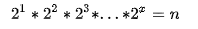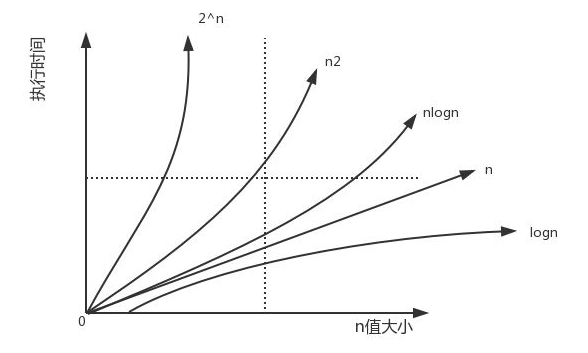# 重学数据结构与算法系列：时间复杂度与空间复杂度``````1 public static int calculator(int n) {
2   int sum = 0;
3   for (int i = 1; i <= n; ++i) {
4     for (int j = 1; j <= n; ++j) {
5     sum = sum + j;
6           }
7   }
8   return sum;
9 }``````

## 1、O（1）

``````1 int a = 1；
2 int b = 2;
3 int result = a * b;``````

## 2、O（n）

``````1 public static int calculator(int n) {
2  int sum = 0;
3  for (int i = 1; i <= n; ++i) {
4    sum = sum + i;
5  }
6  return sum;
7 }``````

## 3、O（n²）

`````` public static int calculator(int n) {
int sum = 0;
for (int i = 1; i <= n; ++i) {
for (int j = 1; j <= n; ++j) {
sum = sum + j;
}
}
return sum;
}``````

## 4、O（logn）

``````1 public static int calculator(int n) {
2  i=1;
3  while (i <= n) {
4      i = i * 2;
5  }
6  return i;
7 }````````````1 public static void save(int n) {
2    Set<Integer> ds = new HashSet<>(n);
3    for (int i = 0; i < n; i++) {
5    }
6 }``````（图片来源于网络）

-- 展开阅读全文 --

KillDefender 的 Beacon 对象文件 PoC 实现
« 上一篇 02-09
Web安全—逻辑越权漏洞（BAC）